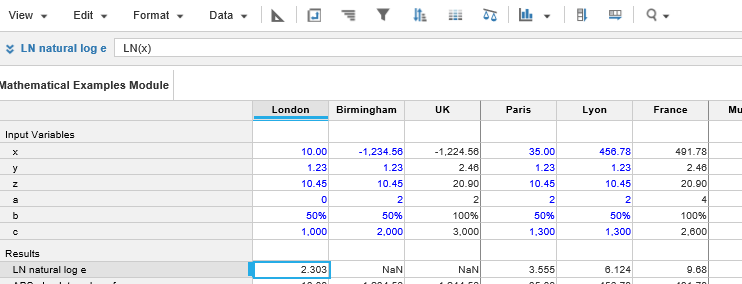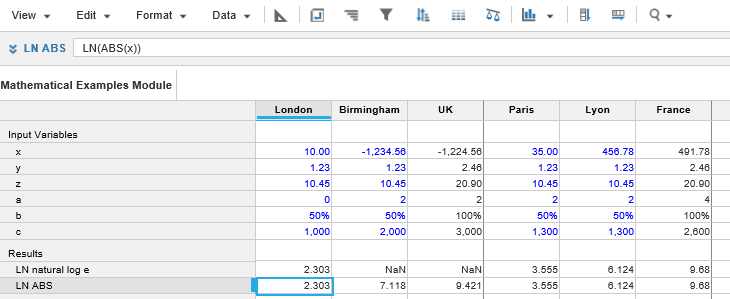1. Calculation functions
2. All Functions
3. Numeric Functions
4. LNReturns the natural logarithm of a number, based on the constant e. Natural logarithms are frequently used to calculate the rate of growth.

This function is the inverse of the EXP function, which raises e to the nth power.

## Syntax

`LN(x) `

where:

• x: Number to return the natural logarithm for

## Format

Input Format Output Format

x: Number

Number

## Arguments

The function uses the following arguments:

• x: Number: Numeric line item, property, or expression

## Constraints

The function has the following constraints:

• LN can only be used with positive numbers

## Example

In the example below, the line item LN natural log e shows the result of the formula:

`LN(x)`Note: Negative numbers display as NaN. You can use the ABS function to remove the negative value to return a numerical result, as shown below.
`LN(ABS(x))`